Backfitting and Local Scoring Algorithms

Much of the development and notation in this section follows Hastie and Tibshirani (1986).

Consider the estimation of the smoothing termsin the additive model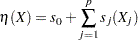where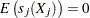for every. Since the algorithm for additive models is the basis for fitting generalized additive models, the algorithm for additive models is discussed first.

Many ways are available to approach the formulation and estimation of additive models. The backfitting algorithm is a general algorithm that can fit an additive model with any regression-type fitting mechanisms.

Define theth set of partial residuals as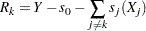then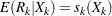. This observation provides a way to estimate each smoothing functiongiven estimates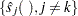for all the others. The resulting iterative procedure is known as the backfitting algorithm (Friedman and Stuetzle 1981). The following formulation is taken from Hastie and Tibshirani (1986).

### The Backfitting Algorithm

The unweighted form of the backfitting algorithm is as follows:

1. Initialization: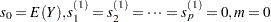2. Iterate:;
fortodo:;;

3. Until:fails to decrease, or satisfies the convergence criterion.

In the preceding notation,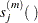denotes the estimate of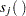at theth iteration. It can be shown that with many smoothers (including linear regression, univariate and bivariate splines, and combinations of these), RSS never increases at any step. This implies that the algorithm always converges (Hastie and Tibshirani, 1986). Note, however, that for distributions other than Gaussian, numerical instabilities with weights can cause convergence problems. Even when the algorithm converges, the individual functions need not be unique, since dependence among the covariates can lead to more than one representation for the same fitted surface.

A weighted backfitting algorithm has the same form as for the unweighted case, except that the smoothers are weighted. In PROC GAM, weights are used with non-Gaussian data in the local scoring procedure described later in this section.

The GAM procedure uses the following condition as the convergence criterion for the backfitting algorithm: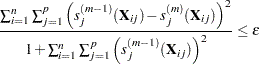where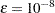by default; you can change this with the EPSILON=  option in the MODEL statement.

The algorithm described so far fits only additive models. The algorithm for generalized additive models is a little more complicated. Generalized additive models extend generalized linear models in the same manner that additive models extend linear regression models—that is, by replacing the formwith the additive form. See Generalized Linear Models Theory in Chapter 39, The GENMOD Procedure, for more information.

PROC GAM fits generalized additive models by using a modified form of adjusted dependent variable regression, as described for generalized linear models in McCullagh and Nelder (1989), with the additive predictor taking the role of the linear predictor. Hastie and Tibshirani (1986) call this the local scoring algorithm. Important components of this algorithm depend on the link function for each distribution, as shown in the following table.

 Distribution Link Adjusted Dependent () Weights () Normal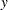1 Binomial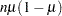Gamma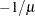Poisson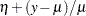Inverse Gaussian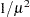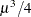Once the distribution and hence these quantities are defined, the local scoring algorithm proceeds as follows.

### The General Local Scoring Algorithm

1. Initialization: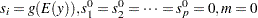2. Iterate:;
Form the predictor, mean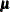, weights, and adjusted dependent variablebased on their corresponding values from the previous iteration: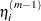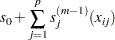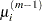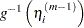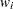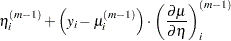where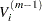is the variance of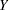at. Fit an additive model toby using the backfitting algorithm with weightsto obtain estimated functions;

3. Until:
The convergence criterion is satisfied or the deviance fails to decrease. The deviance is an extension to generalized linear models of the RSS; see Goodness of Fit in Chapter 39, The GENMOD Procedure, for a definition.

The GAM procedure uses the following condition as the convergence criterion for local scoring: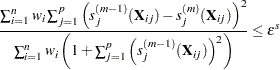whereby default; you can change this with the EPSSCORE= option in the MODEL statement.

The estimating procedure for generalized additive models consists of two loops. Inside each step of the local scoring algorithm (outer loop), a weighted backfitting algorithm (inner loop) is used until convergence or until the RSS fails to decrease. Then, based on the estimates from this weighted backfitting algorithm, a new set of weights is calculated and the next iteration of the scoring algorithm starts. The scoring algorithm stops when the convergence criterion is satisfied or the deviance of the estimates stops decreasing.